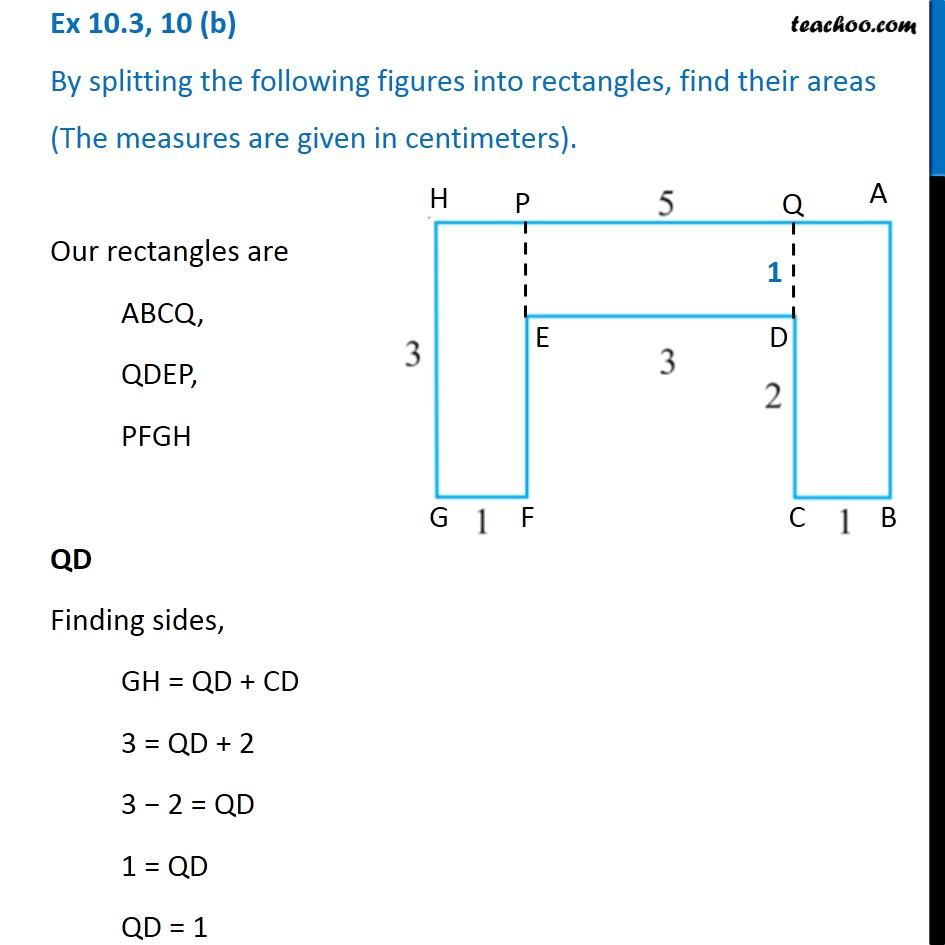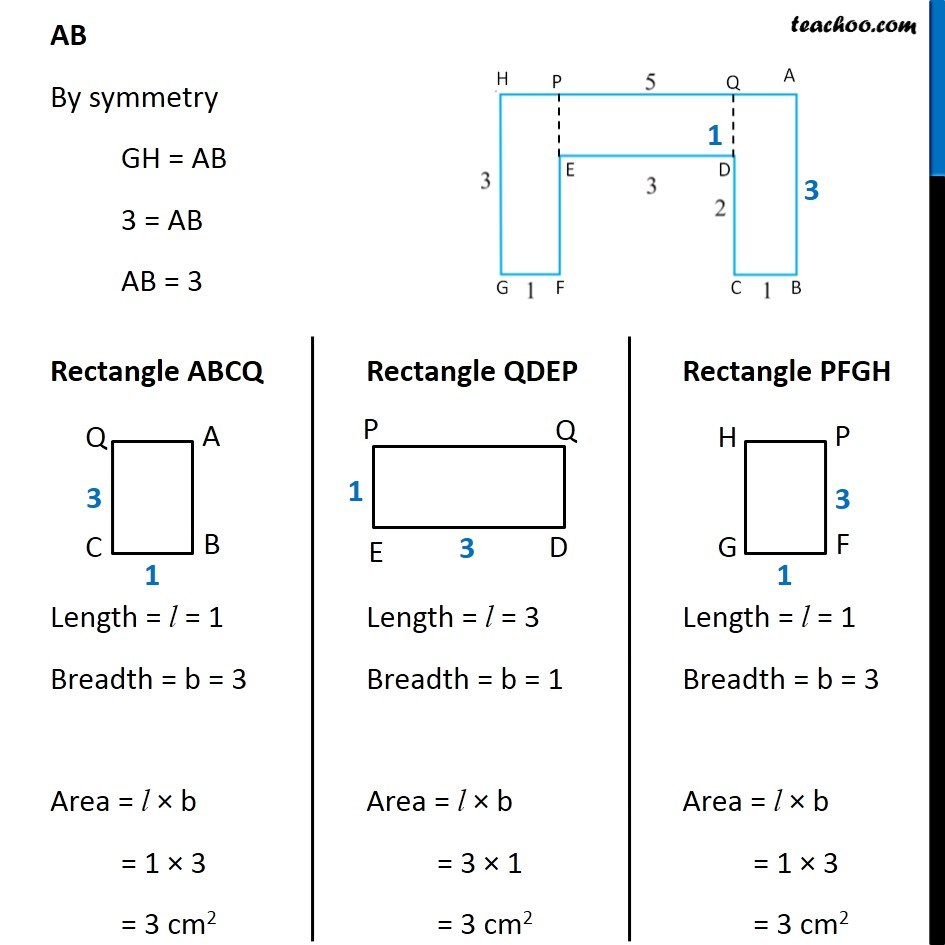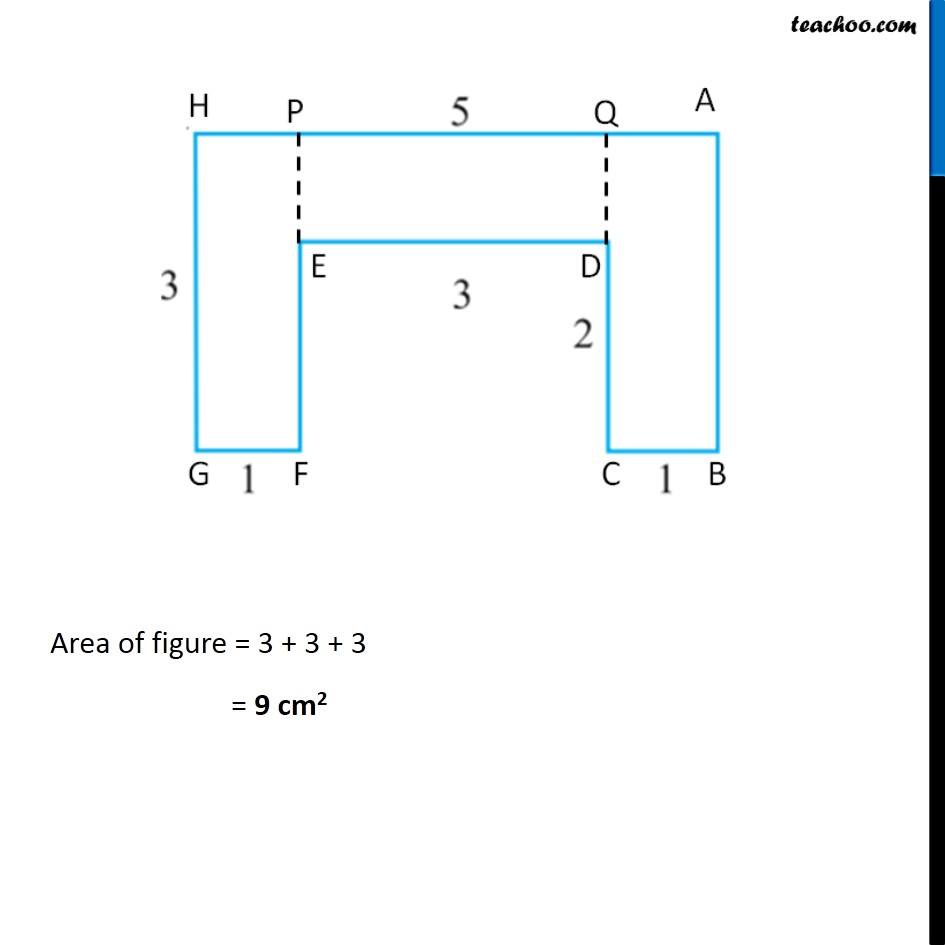Ex 10.3

Chapter 10 Class 6 Mensuration
Serial order wiseGet live Maths 1-on-1 Classs - Class 6 to 12

### Transcript

Ex 10.3, 10 (b) By splitting the following figures into rectangles, find their areas (The measures are given in centimeters). Our rectangles are ABCQ, QDEP, PFGH QD Finding sides, GH = QD + CD 3 = QD + 2 3 − 2 = QD 1 = QD QD = 1 AB By symmetry GH = AB 3 = AB AB = 3 Rectangle ABCQ Length = l = 1 Breadth = b = 3 Area = l × b = 1 × 3 = 3 cm2 Rectangle QDEP Length = l = 3 Breadth = b = 1 Area = l × b = 3 × 1 = 3 cm2 Rectangle PFGH Length = l = 1 Breadth = b = 3 Area = l × b = 1 × 3 = 3 cm2 Area of figure = 3 + 3 + 3 = 9 cm2